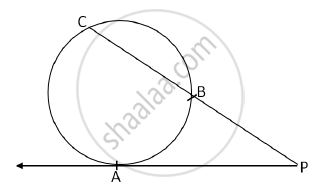# In the following figure, a tangent segment PA touching a circle in A and a secant PBC is shown. If AP = 15, BP = 10, find BC - Geometry

In the following figure, a tangent segment PA touching a circle in A and a secant PBC is shown. If AP = 15, BP = 10, find BC.#### Solution

PA is a tangent segment and PBC is the secant.

therefore PB xx PC=PA^2 ........(Relationship to Tangent-Secant Theorem)

therefore 10xxPC=15^2

PC=225/10=22.5

Now , PB+BC=PC

therefore 10+BC=22.5

therefore BC=22.5-10= 12.5

Concept: Tangent - Secant Theorem
Is there an error in this question or solution?
2015-2016 (March) Set A

Share